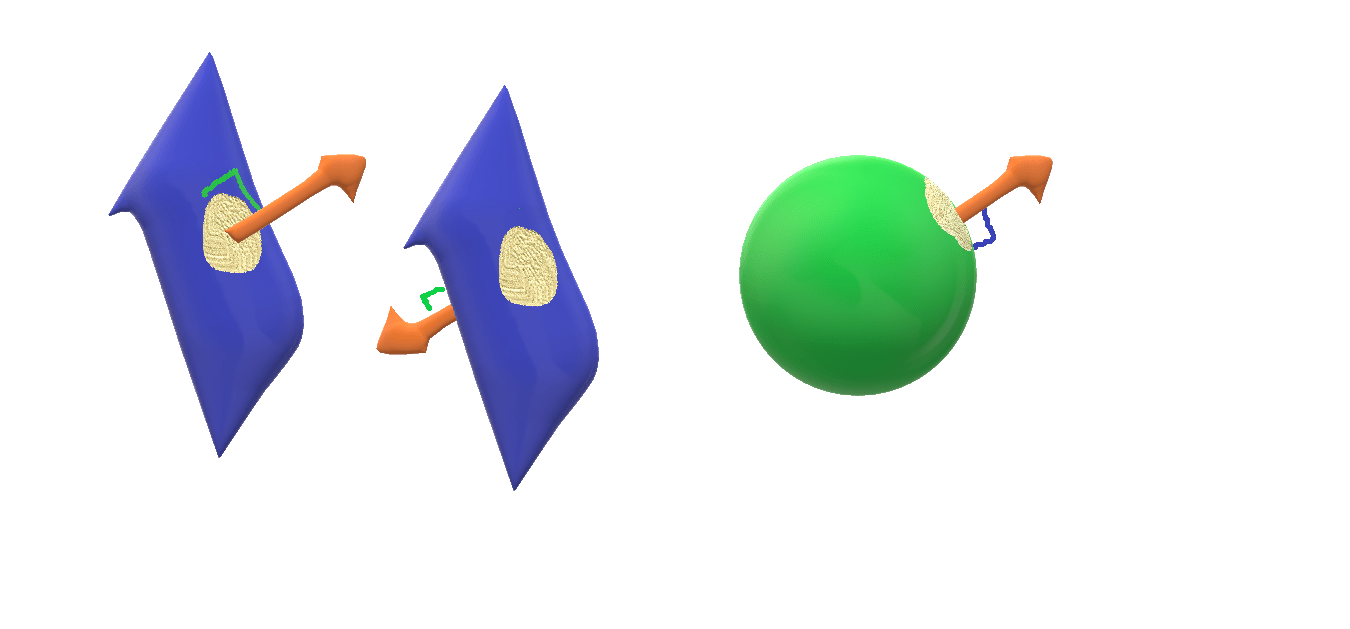#### Area Vector

2

Ohh! Finally you are here and that means you are interested in knowing about area vector! So the question arises, ” Is area a vector?” and the answer is no, of course not. Actually area is a physical quantity that don’t have properties of vector, so it is scalar.

“ The conclusion is area is a scalar. “

So now another question comes into role…if area is scalar why are we here….here discussing about area vector. And the answer is simple. In Physics there are many situations where treating area as vector simplifies mathematical formulations and calculations. Note the word I am using here ‘treating’ i.e. area is still a scalar and not vector. We are only associating a hypothetical vector with area for mathematical ease. And that’s it.

So, now let’s try to understand the facts and assumptions regarding this ( area vector – though I don’t like to say it vector ) vector. Area is always associated with some surface. Surface can be open or closed. Depending on this there are two scenarios, but before going too far let’s consider a general assumption-

While thinking of a vector we think of two things – very first one is magnitude and second but most important one is direction ( If you don’t know about vectors, I would recommend reading my article named The Vectors ). So let’s consider these two aspects. The magnitude of area vector is always ( in both of the scenarios ) taken to be equal to area of the surface. But while determining direction we need to be extra careful. This is the case where two scenarios ( open surface and closed surface ) varies. So let’s discuss it under two headings –

## For Open Surface :

While assuming area vector for open surface, the direction is always chosen to be normal to the surface. When you actually go to draw a normal to any surface you will notice there are two possibilities for drawing normal. It could be inwards or outwards. And you are free to chose any of these two directions as the direction of area vector.

## For Closed Surface :

Unlike in the case of open surface, The direction of area vector is always chosen outwards, and this is convention. So we are not free while choosing direction for area vector in case of closed surface.

2
Tags